• 在 Narrow Phase 中，SAT（Separating Axis Theorem，分离轴定理）碰撞检测算法直观且高效。然而，它只适用于 凸多边形 的碰撞检测。它的原理清晰易懂，即 若两个物体没有发生碰撞，则总会存在一条直线，能将两个...
原理
概述
碰撞检测可分为 Broad Phase （粗略检测）与 Narrow Phase （精细检测） 两个阶段。在 Narrow Phase 中，SAT（Separating Axis Theorem，分离轴定理）碰撞检测算法直观且高效。然而，它只适用于 凸多边形 的碰撞检测。它的原理清晰易懂，即 若两个物体没有发生碰撞，则总会存在一条直线，能将两个物体分离 。于是，我们把这条能够隔开两个物体的线称为 分离轴 ，如下图：我们可以很直观的看到，左边的图形总是会存在一条直线，将未碰撞的两个物体分离；而右边的图形，我们没能够找到一条轴线，将两个图形分开。那么，要如何处理右边碰撞的情况呢？
多边形与多边形
投影
为了便于理解，我们先从矩形开始分析。假设我们有两个矩形，已知矩形的顶点坐标，并且两个矩形发生了碰撞，如下图所示：根据分离轴定理，我们似乎没能找到一条轴线能够将两个矩形分离。想要让计算机知道没有这一条轴线，我们需要进行 投影，即将两个图形各自的顶点都往 X 轴和 Y 轴进行投影：此时，仍无法直观的感受到分离轴的存在。其实将两个矩形分开后，就可以找到一条能将两个图形分开的轴线：注意我们的两个矩形在X轴上的投影点，很清晰的可以看到，两个矩形被这条黑色的轴线分隔开。
与此同时，他们的 投影点 也被这条轴线分隔开，如图中的 $P$ 点与 $I$ 点。将紫色的矩形命名为 $A$ ，绿色的矩形命名为 $B$ 。于是，他们在  $X$  轴投影点的最大值与最小值所构成的区间，可以这样表示：
${ projection_A | projection_A \in \left[ 1, 3 \right] } \\\ { projection_B | projection_B \in \left[ 4, 7 \right] }$
分离轴定理的分离轴，分离的是两个矩形的投影点集合。简而言之，就是指 两个投影点集合的交集是否为空集 。如果是空集，则存在一条轴，将两个投影点集分隔开，如果是非空集合，则找不到这样的轴。如果在   $X$   和   $Y$  轴，两个矩形的投影点集合的交集 全都是非空集合 ，那么，我们可以判定两个矩形发生碰撞。
这只是一部分，针对于多边形的投影，我们可以将两个多边形的顶点投影在 多边形每条边的中垂线上 ，获得投影点的集合后再进行比较。
首先以蓝色多边形的  $AB$  边开始，做出中垂线，标出每个顶点的投影点，并对投影集合的最大点和最小点进行颜色标记，如下图：隐藏垂线，尝试连接  $A'D'$  与  $E'G'$ ，构成的直线视为投影点集合，如下图：可以很明显的看到两个线段 相交，于是我们认为，两个多边形在  $AB$  边上是发生碰撞的。
于是，我们将两个多边形的所有边进行如上操作，并且在两个线段相交的情况下，以红色线段标记他们重叠的部分，如下图：从上图可以看出，每一个投影都存在红色的重叠部分，意味着可以判断两个多边形 发生碰撞。而我们所标记的红色重叠线段中，长度最小 线段，称为 穿透长度，如果设置一个起点和终点，构成有大小和方向的矢量，则称为 穿透矢量（Penetration Vector）或 分离向量。
将这些红色线段排序，即可得到最小的线段为  $CD$ 边投影 的  $E'C'$  。将  $E'$  设为起点，  $C'$  设为终点，向量  $\vec {E'C'}$  为黄色多边形的分离向量。将黄色多边形的每个顶点坐标叠加上穿透向量，则可将两个物体分离：多边形与圆
投影
与多边形之间的碰撞大致相同，然而圆有一条 动轴，它由圆心与 离圆最近的多边形顶点 连接而成。找到后，分析办法仍与多边形之间的碰撞类似：其中的  $DH$  线段就是重叠部分，之后寻找最短的线段，与上一部分同样的处理手段。


展开全文物理引擎
• 直观来看，如果两个凸多面体不相交，那么必定存在一个空间平面，使得这两个多面体分别位于平面两侧。如果找不到这样的平面，那么可以判定其相交。这个情况等价于：如果能找到垂直于某个平面的...SAT是一种检测多边形
        直观来看，如果两个凸多面体不相交，那么必定存在一个空间平面，使得这两个多面体分别位于平面两侧。如果找不到这样的平面，那么可以判定其相交。这个情况等价于：如果能找到垂直于某个平面的直线，使得多面体在这条直线上的投影不相交，那么就可以判定多面体不相交；否则就相交。这条直线，就是分离轴。一般而言，检验多面体在直线上的投影，会比检验多面体是否位于平面两侧更加方便。

SAT是一种检测凸多边形相交的算法，他的内容是，如果能找到一条轴，使得两个物体在该轴上的投影互不重叠，那么这两个物体就是不相交的。

原理很容易理解，这个算法的关键在于，如何找到这条轴。 在2D的情况下，两个多边形每条边的法向量包含了这条轴的所有可能性。所以我们只需要枚举两个多边形的每条边的法向量即可。

2D向量的法向量非常好求 向量（X,Y）的法向量为（Y,-X）或（-Y,X）（设待求斜率为k，根据垂直的向量斜率之积等于-1，Y/X*k= - 1  =>  k=  - X/Y），这个算法不需要考虑方向，所以任选一种即可。然后分别计算这两个多边形的所有点在此向量上的投影，并求出最大最小区域，如果没有重合，那么直接确定这两个多边形不重合，如果有重叠，那么继续判断下一条边的法向量。

展开全文• 在游戏开发中，我们一般常用的碰撞检测算法有AABB，OBB以及分离轴算法。 AABB与OBB一般用于矩形的碰撞检测，而多边形的碰撞检测一般使用分离轴算法，这里说的都是2D图形的碰撞检测 一、什么是分离轴？ 将两个多边形...
在游戏开发中，我们一般常用的碰撞检测算法有AABB，OBB以及分离轴算法。
AABB与OBB一般用于矩形的碰撞检测，而多边形的碰撞检测一般使用分离轴算法，这里说的都是2D图形的碰撞检测

一、什么是分离轴？

将两个多边形投影到任意的一条轴上，如果能找到至少有一个轴上的两个多边形投影没有重叠，那么就说明这两个多边形没有发生碰撞。
在程序实现中，我们不可能去检测所有的轴，这是不现实的，不过好在多边形的特性，我们只需要以多边形的每一条边的法线为轴，分别检测一遍就好了。
分离轴适用的是凸多边形之间检测，不适用于凹多边形，凹多边形的检测，可以通过算法将凹多边形分割成多个凸多边形再进行计算。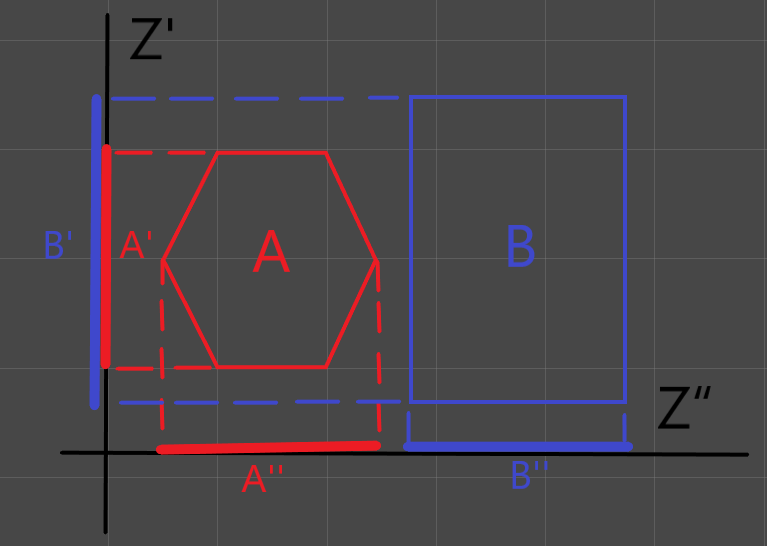分离轴.png

多边形A,B在轴Z''上的投影A''与B''是分离的，所以多边形A,B是分离的。

二、算法实现

2.1、多边形与多边形

（1）辅助方法

---获取两点的距离
function GetDis(vec1,vec2)
local x1 = vec1.x or vec1
local y1 = vec1.y or vec1
local x2 = vec2.x or vec2
local y2 = vec2.y or vec2

local disX = x1 - x2
local disY = y1 - y2
local dis = math.sqrt(disX * disX + disY * disY)
return dis
end

---向量归一化
function Normalize(vec)
local x = vec or vec.x
local y = vec or vec.y
local mag = math.sqrt(x*x + y*y)
if type(vec) == "table" then
vec = x/mag
vec = y/mag
end
vec.x = x/mag
vec.y = y/mag
end

---点乘
function Dot(vec1,vec2)
local x1 = vec1.x or vec1
local y1 = vec1.y or vec1
local x2 = vec2.x or vec2
local y2 = vec2.y or vec2
return x1*x2 + y1*y2
end

---精确到小数点后n位
---num 浮点数
---n 浮点数精确位数
function FloatAccurateN(num,n)
if type(num) ~= "number" then
return num;
end
n = n or 0;
n = math.floor(n)
if n < 0 then
n = 0;
end
local nDecimal = 10 ^ n
local nTemp = math.floor(num * nDecimal);
local nRet = nTemp / nDecimal;
return nRet;
end

---二维向量的向量积
---大小的绝对值表示两个向量构成的三角形的面积的2倍
---正负表示与两个向量构成的平面的法线的方向
function VectorProduct(vec1,vec2)
local vec1X = vec1.x or vec1
local vec1Y = vec1.y or vec1
local vec2X = vec2.x or vec2
local vec2Y = vec2.y or vec2
return vec1X*vec2Y - vec2X*vec1Y
end

function Add(pt1,pt2)
return {x = pt1.x + pt2.x , y = pt1.y + pt2.y }
end

function Sub(pt1,pt2)
return {x = pt1.x - pt2.x , y = pt1.y - pt2.y }
end

-- 计算圆周上的点位置
-- 返回的是通过圆心与水平夹角为angleRadians度的直径在圆上的两个交点
function CalCirclePos(centerPos, radius, angleRadians)
return Add(centerPos, {x=math.cos(angleRadians)*radius, y=math.sin(angleRadians)*radius}),Sub(centerPos, {x=math.cos(angleRadians)*radius, y=math.sin(angleRadians)*radius})
end

---将角度转换为逆时针角度
---rotation (0 ~ 180逆时针,0 ~ -180顺时针)
function changeRotationToInverse(rotation)
rotation = rotation or 0
if rotation < 0 then
rotation = rotation + 360
end
return rotation or 0
end


（2）创建多边形

--创建一个多边形
--name:多边形名字
--offset:实际点与多边形的偏移量，offset为多边形的原点，多边形的顶点位置都是相对于这个点的偏移量
--vertices ： 多边形的顶点数组，位置相对于offset
--rotation ： 旋转角度（角度不是弧度（0~180为逆时针，0~-180为顺时针）
function Polyon(name,offset,vertices,rotation)
local polyon = {}
polyon.name = name or "polyon"
polyon.offset = {offset.x or offset or 0,offset.y or offset or 0}
-- 弧度
polyon.rotation = math.rad(changeRotationToInverse(rotation))
--- 模板顶点，相对于offset为原点的顶点数组
polyon._tempVertices = {}
for i, vertice in ipairs(vertices) do
local x = vertices[i]
local y = vertices[i]
table.insert(polyon._tempVertices,{x=x,y=y})
end
--顶点数组,实际顶点坐标
polyon._vertices = {}
-- 平面中，一个点(x,y)绕任意点(dx,dy)逆时针旋转a度后的坐标
-- xx= (x - dx)*cos(a) - (y - dy)*sin(a) + dx ;
-- yy= (x - dx)*sin(a) + (y - dy)*cos(a) +dy ;
for i, vertice in ipairs(vertices or {}) do
local x = (vertices[i]*math.cos(polyon.rotation))-(vertices[i]*math.sin(polyon.rotation))
local y = (vertices[i]*math.sin(polyon.rotation))+(vertices[i]*math.cos(polyon.rotation))
table.insert(polyon._vertices,{x=x,y=y})
end

---边
polyon._edges = {}
for i=1,#polyon._vertices do
table.insert(polyon._edges, createSegment(polyon._vertices[i], polyon._vertices[1+i%(#polyon._vertices)]))
end

polyon.centerPoint = {x=0,y=0}

--- 注册点到中心点的距离
polyon._centerToAnchorDistance = GetDis({0,0},polyon.offset)
--- 中心点相对于注册点的旋转弧度
polyon._centerToAnchorRadian = math.atan2(polyon.offset, polyon.offset)

return polyon
end

---多边形的边
---@param vertice1 第一个顶点
---@param vertice2 第二个顶点
function createSegment(vertice1,vertice2)
-- dir为多边形的边的向量
local segment = {pointA = vertice1,pointB = vertice2,dir = {vertice2.x - vertice1.x,vertice2.y - vertice1.y}}
return segment
end

-- 打印多边形
function PrintPolygon(polyon)
print(polyon.name.." offset ",polyon.offset,polyon.offset)
print(polyon.name.." centerPoint ",polyon.centerPoint.x,polyon.centerPoint.y)
print(polyon.name.."模板顶点信息：===========================================")
for index, value in ipairs(polyon._tempVertices) do
print(string.format("x = %f, y = %f",polyon._tempVertices[index].x,polyon._tempVertices[index].y))
end
print(polyon.name.."模板顶点信息：===========================================")
print(polyon.name.."实际顶点信息：===========================================")
for index, value in ipairs(polyon._vertices) do
print(string.format("x = %f, y = %f",polyon._vertices[index].x,polyon._vertices[index].y))
end
print(polyon.name.."实际顶点信息：=======================================")
print(polyon.name.."边信息：===========================================")
for index, value in ipairs(polyon._edges) do
print(string.format("dir = (%f,%f)",polyon._edges[index].dir,polyon._edges[index].dir))
end
print(polyon.name.."边信息：=======================================")
end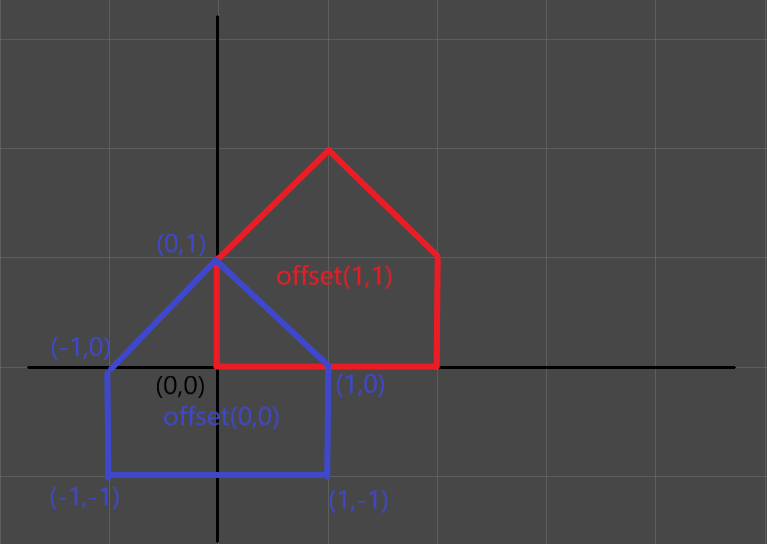多边形offset.png

上图为两个顶点相同，offset不同的两个多边形在（0,0）点位置。

（3）设置多边形

---设置多边形的实际位置，更新多边形的信息
---polyon 多边形
---x 碰撞体的实际位置X
---y 碰撞体的实际位置y
---rotation 是角度不是弧度
function setPolyon(polyon, x, y, rotation)
rotation = changeRotationToInverse(rotation) or 0
local r = math.rad(rotation)
polyon.rotation = r
---相对于世界坐标系旋转的弧度
local radian = polyon._centerToAnchorRadian + r
local dx = polyon._centerToAnchorDistance * math.cos(radian)
local dy = polyon._centerToAnchorDistance * math.sin(radian)
---中心点（offset点）的世界坐标
polyon.centerPoint.x = x + dx
polyon.centerPoint.y = y + dy

---更新多边形顶点位置（相对于世界坐标的）
for i, vertice in ipairs(polyon._vertices) do
local x = polyon._tempVertices[i].x
local y = polyon._tempVertices[i].y
-- 平面中，一个点(x,y)绕任意点(dx,dy)逆时针旋转a度后的坐标
-- xx= (x - dx)*cos(a) - (y - dy)*sin(a) + dx ;
-- yy= (x - dx)*sin(a) + (y - dy)*cos(a) +dy ;
polyon._vertices[i].x = polyon.centerPoint.x + (x*math.cos(polyon.rotation))-(y*math.sin(polyon.rotation))
polyon._vertices[i].y = polyon.centerPoint.y + (x*math.sin(polyon.rotation))+(y*math.cos(polyon.rotation))
end

---更新边的信息
for i=1,#polyon._vertices do
local pointA = polyon._vertices[i]
local pointB = polyon._vertices[1+i%(#polyon._vertices)]
polyon._edges[i].pointA = pointA
polyon._edges[i].pointB = pointB
polyon._edges[i].dir = pointB.x - pointA.x
polyon._edges[i].dir = pointB.y - pointA.y
end
end


先计算中心点相对于实际坐标（x,y）的位置，计算各个顶点在多边形旋转以后与中心点的相对位置，计算出各个顶点的实际坐标点，通过各个顶点的实际坐标再更新多边形的边的信息

（5）计算多边形碰撞

---polygonA 多边形
---polygonB 多边形
---分离轴，以多边形的每条边的法向量为轴，将多边形投影到轴上
---如有任意一个轴上的两个多边形的投影不相交，那么这两个多边形就是分离的
function detectorPolygonvsPolygon(polygonA, polygonB)
local aProjection = {}
local bProjection = {}
local segmentNormal  = {}
--遍历多边形的边，以每条边的法线向量作为两个多边形计算投影的投影轴
for i, segment in ipairs(polygonA._edges) do
---边的法线向量
segmentNormal = segment.dir
segmentNormal = -segment.dir
---两个多边形在当前轴上的投影
aProjection,aProjection = getProjectionWithAxis(polygonA,segmentNormal)
bProjection,bProjection = getProjectionWithAxis(polygonB,segmentNormal)

if not projectionContains(aProjection,bProjection) then
return false
end
end

for i, segment in ipairs(polygonB._edges) do
---边的法线向量
segmentNormal = segment.dir
segmentNormal = -segment.dir
---两个多边形在当前轴上的投影
aProjection,aProjection = getProjectionWithAxis(polygonA,segmentNormal)
bProjection,bProjection = getProjectionWithAxis(polygonB,segmentNormal)
if not projectionContains(aProjection,bProjection) then
return false
end
end
return true
end

---计算多边形在轴上的投影
---polyon 多边形
---axis 投影的轴
---返回值为投影两端的最大值与最小值
function getProjectionWithAxis(polyon,axis)
--将投影轴归一化
Normalize(axis)
---在轴上面的投影
local min = Dot(polyon._vertices,axis)
local max = min
for i,v in ipairs(polyon._vertices) do
local proj =  Dot(v, axis)
if proj < min then
min = proj
end
if proj > max then
max = proj
end
end
--返回最小值与最大值，保留小数点后三位
return FloatAccurateN(min,3),FloatAccurateN(max,3)
end

---判断两条投影轴是否相交
function projectionContains(a,b)
local aMin = a
local aMax = a
local bMin = b
local bMax = b
--确保a,b第一个元素小于第二个元素
if aMax < aMin then
aMin = aMax
aMax = a
end
if bMax < bMin then
bMin = bMax
bMax = b
end
return not (aMin > bMax or aMax < bMin)
end


两个多边形分别遍历他们的边，取边的法线向量作为投影轴，将两个多边形分别投影到投影轴上，如果两个多边形的投影不相交，那么两个多边形则没有碰撞。如果所有的边的投影都相交的话，那么两个多边形则发生了碰撞。

（6）计算多边形碰撞测试数据

local polyonA = Polyon("polyonA",{1,1},{{0,1},{-1,0},{-1,-1},{1,-1},{1,0}},0)
setPolyon(polyonA,0,0,0)
local polyonB = Polyon("polyonB",{1,1},{{0,1},{-1,0},{-1,-1},{1,-1},{1,0}},0)
setPolyon(polyonB,2,0,0)
print("多边形A,B碰撞 ： ",detectorPolygonvsPolygon(polyonA,polyonB))

多边形A,B碰撞 ：  true
[Finished in 0.1s]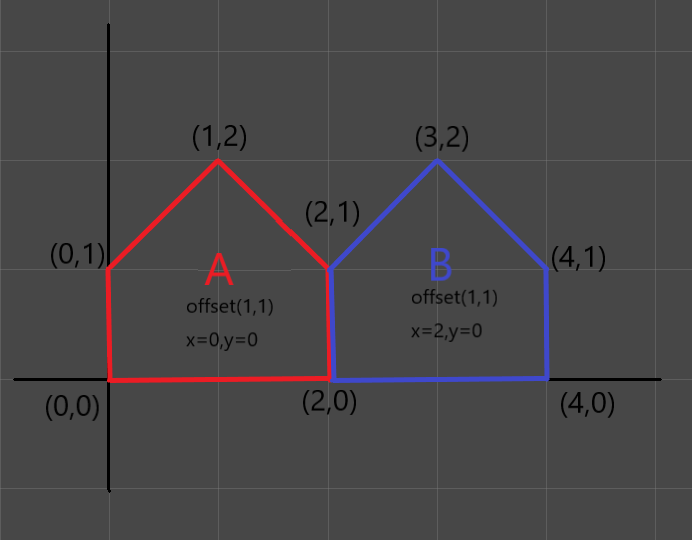平移碰撞1.png

local polyonA = Polyon("polyonA",{0,0},{{0,1},{-1,0},{-1,-1},{1,-1},{1,0}},0)
setPolyon(polyonA,0,0,60)
local polyonB = Polyon("polyonB",{0,0},{{0,1},{-1,0},{-1,-1},{1,-1},{1,0}},0)
setPolyon(polyonB,2.5,0,-30)
print("多边形A,B碰撞 ： ",detectorPolygonvsPolygon(polyonA,polyonB))

多边形A,B碰撞 ：  false
[Finished in 0.1s]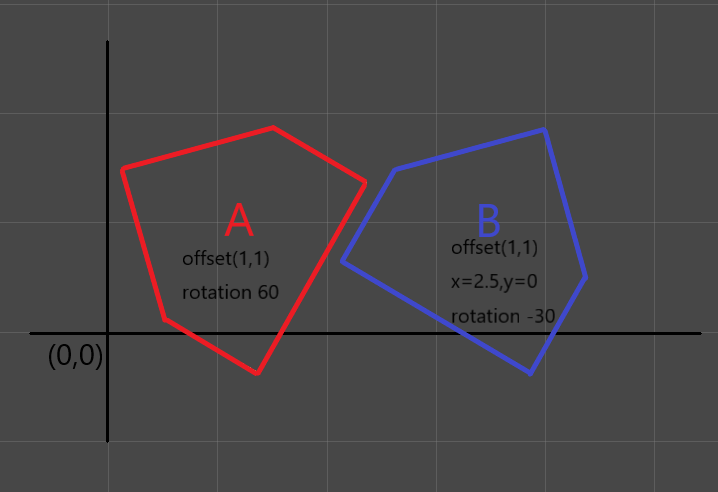旋转检测.png

2.2、多边形与圆

（1）创建圆

---offset:圆心相对于实际位置的偏移点
---radius:圆的半径
function Circle(offset,radius)
local Circle = {}
Circle.radius = radius or 0
Circle.offset = {x=offset.x and offset or 0, y=offset.y and offset or 0}
Circle.centerPoint = {x=0,y=0}
return Circle
end


（2）设置圆的位置

function CircleSet(circle,x,y,radius)
---圆心的实际位置
circle.centerPoint.x = x + circle.offset.x
circle.centerPoint.y = y + circle.offset.y
circle.radius = radius or circle.radius
end


（3）圆与多边形的碰撞

---计算圆形在轴上的投影
---axis 投影的轴
---返回投影两端的最大值与最小值
function getClicleProjectionWithAxis(circle,axis)
Normalize(axis)
---线段的夹角
local rad = math.atan2(axis.y, axis.x)
---经过圆心，线段与圆相交的两个点的位置
local pointInCircle1,pointInCircle2 = CalCirclePos(circle.centerPoint,circle.radius,rad)
---分别两个点在轴上的投影
local min = Dot(pointInCircle1,axis)
local max = min
local min2 = Dot(pointInCircle2,axis)
if min2 < min then min = min2 end
if min2 > max then max = min2 end
return FloatAccurateN(min,3),FloatAccurateN(max,3)
end

---polygon 多边形
---circle 圆形
---原理与多边形判断一样
function detectorPolygonvsCircle(polygon,circle)
local aProjection = {}
local bProjection = {}
local circleCenter = circle.centerPoint
for i, segment in ipairs(polygon._edges) do
---顶点与圆心的连线(轴)
local axes = {segment.dir,-segment.dir}
---多边形在当前轴上的投影
aProjection,aProjection = getProjectionWithAxis(polygon,axes)
---圆在当前轴上的投影
bProjection,bProjection = getClicleProjectionWithAxis(circle,axes)
if not projectionContains(aProjection,bProjection) then
return false
end
end
return true
end


（4）多边形与圆碰撞测试数据

local polyonA = Polyon("polyonA",{1,1},{{0,1},{-1,0},{-1,-1},{1,-1},{1,0}},0)
setPolyon(polyonA,0,0,0)
local circleA = Circle({0,0},1)
CircleSet(circleA,2,0,1)
print("多边形A与圆B碰撞 ： ",detectorPolygonvsCircle(polyonA,circleA))

多边形A与圆B碰撞 ：     true
[Finished in 0.0s]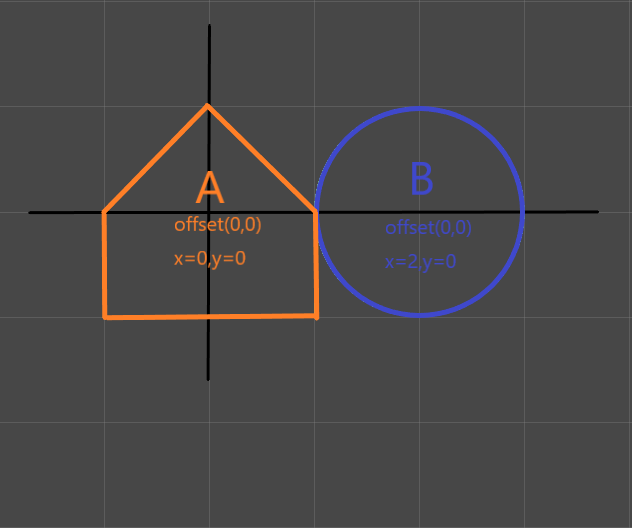多边形与圆平移.png

local polyonA = Polyon("polyonA",{0,0},{{0,1},{-1,0},{-1,-1},{1,-1},{1,0}},0)
setPolyon(polyonA,0,0,-45)
local circleA = Circle({0,0},1)
CircleSet(circleA,2,0,1)
print("多边形A与圆B碰撞 ： ",detectorPolygonvsCircle(polyonA,circleA))

多边形A与圆B碰撞 ：     false
[Finished in 0.1s]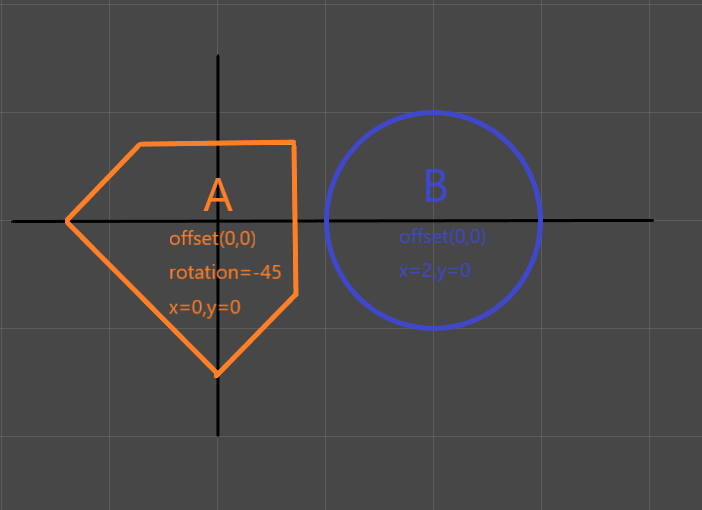多边形与圆的旋转平移.png

2.3、多边形与点

(1) 实现

---多边形与点的碰撞(判断一个点是在多边形内，还是在多边形上，还是在多边形外)
---polygon 多边形
---point 需要检测的点
function detectorPolygonvsPoint(polygon,point)
local pointX = point.x or point
local pointY = point.y or point
local fristvectorproduct = 0
for i, edge in ipairs(polygon._edges) do
local vertice = edge.pointA
---边的第一个顶点point的向量
local vertice2point = {pointX - vertice.x,pointY - vertice.y}
---边与vertice2point的向量积
local vectorproduct = FloatAccurateN(VectorProduct(edge.dir,vertice2point),3)
---点在多边形的边上
if vectorproduct == 0 then
return true
end
if i == 1 then
fristvectorproduct = vectorproduct
else
if fristvectorproduct * vectorproduct < 0 then
return false
end
end
end
return true
end


多边形可看做从某点出发的闭合回路，内部的点永远在回路的同一边。通过边与点的连线的向量积(叉积)的正负表示方向，顺时针方向，所有向量积数值均为负，逆时针方向，所有向量积数值均为正多边形与点参考文章

(2)测试数据

local polyonA = Polyon("polyonA",{0,0},{{0,1},{-1,0},{-1,-1},{1,-1},{1,0}},0)
setPolyon(polyonA,0,0,0)
local pointB = {x=0,y=1}
print("多边形A与点B碰撞 ： ",detectorPolygonvsPoint(polyonA,pointB))

多边形A与点B碰撞 ：     true
[Finished in 0.0s]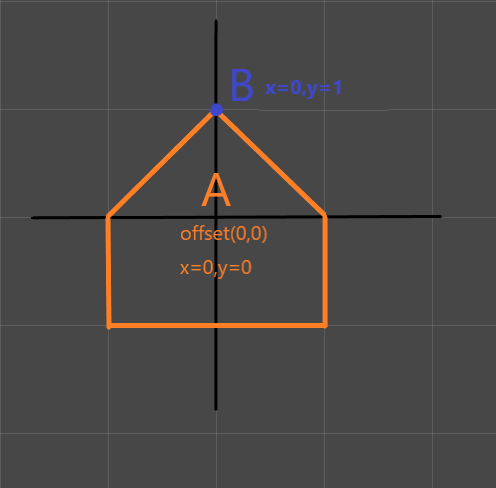多边形与点.png

local polyonA = Polyon("polyonA",{0,0},{{0,1},{-1,0},{-1,-1},{1,-1},{1,0}},0)
setPolyon(polyonA,0,0,30)
local pointB = {x=0,y=1}
print("多边形A与点B碰撞 ： ",detectorPolygonvsPoint(polyonA,pointB))

多边形A与点B碰撞 ：     false
[Finished in 0.0s]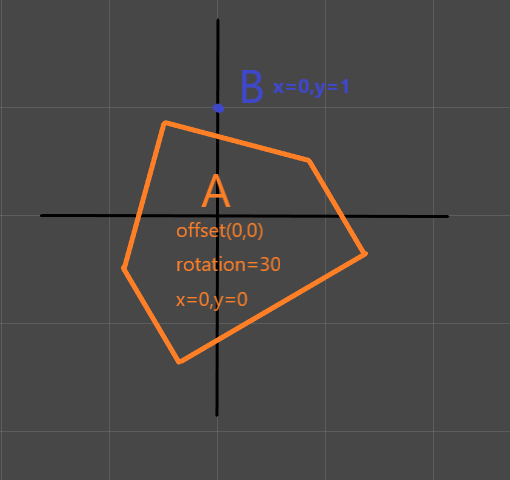多边形与点旋转.png

三、分离轴优缺点

优点：
速度快，算法简单易懂，过程只需要简单的向量运算，只要有一个轴满足不相交，就可以停止检测。
缺点：
只能用于检测凸多边形。只能知道多边形发生了碰撞，但是并不能知道是碰撞的具体信息（碰撞位置），但是可以间接的知道碰撞的深度。

代码下载
提取码: ffpv


展开全文• 多边形碰撞检测在游戏开发中是非常常用的算法，最直接的算法是检测两个多边形的每个点是否被包含，但是由于多边形的数量和多边形点的数量导致这种最直接的算法的效率非常之低。本文将介绍一个非常简单并且效率极高的...
转自: http://www.jianshu.com/p/4000a301c32a

多边形碰撞检测在游戏开发中是非常常用的算法，最直接的算法是检测两个多边形的每个点是否被包含，但是由于多边形的数量和多边形点的数量导致这种最直接的算法的效率非常之低。本文将介绍一个非常简单并且效率极高的算法——“分离轴算法”，并用C语言和Lua语言分别实现该算法，可以分别用于Cocos2d和Corona开发。

分离轴算法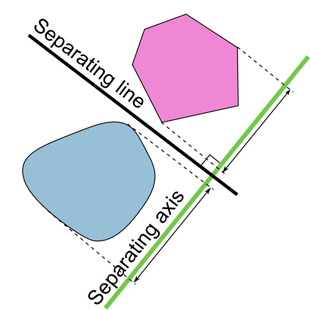上图就是分离轴算法的图示，首先需要知道分离轴算法只适用于“凸多边形”，但是由于“凹多边形”可以分成多个凸多边形组成，所以该算法可以用于所有多边形碰撞检测。不知道凹多边形是什么的看下图：
<!-- more -->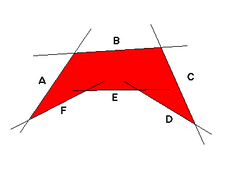凹多边形就是含有顶点的内角度超过180°的多边形，反之就是凸多边形。

简单的说，分离轴算法就是指两个多边形能有一条直线将彼此分开，如图中黑线“Seperating line”，而与之垂直的绿线就是分离轴“Separating axis”。图中虚线表示的是多边形在分离轴上的投影（Projection）。详细的数学理论请查看wiki，我这里只讲该算法的实现方式。如果用伪代码来表示就是：
bool sat(polygon a, polygon b){
for (int i = 0; i < a.edges.length; i++){
vector axis = a.edges[i].direction; // Get the direction vector of the edge
axis = vec_normal(axis); // We need to find the normal of the axis vector.
axis = vec_unit(axis); // We also need to "normalize" this vector, or make its length/magnitude equal to 1

// Find the projection of the two polygons onto the axis
segment proj_a = project(a, axis), proj_b = project(b, axis);

if(!seg_overlap(proj_a, proj_b)) return false; // If they do not overlap, then return false
}
... // Same thing for polygon b
// At this point, we know that there were always intersections, hence the two polygons must be colliding
return true;
}

首先取多边形a的一边，得出该边的法线（即分离轴）。然后算出两个多边形在该法线上的投影，如果两个投影没有重叠则说明两个多边形不相交。遍历多边形a所有的边，如果所有法线都不满足条件，则说明两多边形相交。

算法实现

首先我们需要定义几个数据类型和函数。
Lua:
function vec(x, y)
return {x, y}
end

v = vec -- shortcut

function dot(v1, v2)
return v1*v2 + v1*v2
end

function normalize(v)
local mag = math.sqrt(v^2 + v^2)
return vec(v/mag, v/mag)
end

function perp(v)
return {v,-v}
end

function segment(a, b)
local obj = {a=a, b=b, dir={b - a, b - a}}
obj = obj.dir; obj = obj.dir
return obj
end

function polygon(vertices)
local obj = {}
obj.vertices = vertices
obj.edges = {}
for i=1,#vertices do
table.insert(obj.edges, segment(vertices[i], vertices[1+i%(#vertices)]))
end
return obj
end

vec为矢量或者向量，也可表示点；dot为矢量点投影运算；normalize为求模运算；perp计算法线向量；segment表示线段；polygon为多边形，包括顶点vertices和边edges，所有点的顺序必须按顺时针或者逆时针。如：
a = polygon{v(0,0),v(0,1),v(1,1),v(1,0)}

下面是C语言版的：
typedef struct {float x, y;} vec;

vec v(float x, float y){
vec a = {x, y}; // shorthand for declaration
return a;
}

float dot(vec a, vec b){
return a.x*b.x+a.y*b.y;
}

#include <math.h>
vec normalize(vec v){
float mag = sqrt(v.x*v.x + v.y*v.y);
vec b = {v.x/mag, v.y/mag}; // vector b is only of distance 1 from the origin
return b;
}

vec perp(vec v){
vec b = {v.y, -v.x};
return b;
}

typedef struct {vec p0, p1, dir;} seg;

seg segment(vec p0, vec p1){
vec dir = {p1.x-p0.x, p1.y-p0.y};
seg s = {p0, p1, dir};
return s;
}

typedef struct {int n; vec *vertices; seg *edges;} polygon; // Assumption: Simply connected => chain vertices together

polygon new_polygon(int nvertices, vec *vertices){
seg *edges = (seg*)malloc(sizeof(seg)*(nvertices));
int i;
for (i = 0; i < nvertices-1; i++){
vec dir = {vertices[i+1].x-vertices[i].x, vertices[i+1].y-vertices[i].y};seg cur = {vertices[i], vertices[i+1], dir}; // We can also use the segment method here, but this is more explicit
edges[i] = cur;
}
vec dir = {vertices.x-vertices[nvertices-1].x, vertices.y-vertices[nvertices-1].y};seg cur = {vertices[nvertices-1], vertices, dir};
edges[nvertices-1] = cur; // The last edge is between the first vertex and the last vertex
polygon shape = {nvertices, vertices, edges};
return shape;
}

polygon Polygon(int nvertices, ...){
va_list args;
va_start(args, nvertices);
vec *vertices = (vec*)malloc(sizeof(vec)*nvertices);
int i;
for (i = 0; i < nvertices; i++){
vertices[i] = va_arg(args, vec);
}
va_end(args);
return new_polygon(nvertices, vertices);
}

有了数据类型然后就是算法的判断函数。
Lua：
-- We keep a running range (min and max) values of the projection, and then use that as our shadow

function project(a, axis)
axis = normalize(axis)
local min = dot(a.vertices,axis)
local max = min
for i,v in ipairs(a.vertices) do
local proj =  dot(v, axis) -- projection
if proj < min then min = proj end
if proj > max then max = proj end
end

return {min, max}
end

function contains(n, range)
local a, b = range, range
if b < a then a = b; b = range end
return n >= a and n <= b
end

function overlap(a_, b_)
if contains(a_, b_) then return true
elseif contains(a_, b_) then return true
elseif contains(b_, a_) then return true
elseif contains(b_, a_) then return true
end
return false
end

project为计算投影函数，先计算所有边长的投影，然后算出投影的最大和最小点即起始点；overlap函数判断两条线段是否重合。
C：
float* project(polygon a, vec axis){
axis = normalize(axis);
int i;
float min = dot(a.vertices,axis); float max = min; // min and max are the start and finish points
for (i=0;i<a.n;i++){
float proj = dot(a.vertices[i],axis); // find the projection of every point on the polygon onto the line.
if (proj < min) min = proj; if (proj > max) max = proj;
}
float* arr = (float*)malloc(2*sizeof(float));
arr = min; arr = max;
return arr;
}

int contains(float n, float* range){
float a = range, b = range;
if (b<a) {a = b; b = range;}
return (n >= a && n <= b);
}

int overlap(float* a_, float* b_){
if (contains(a_,b_)) return 1;
if (contains(a_,b_)) return 1;
if (contains(b_,a_)) return 1;
if (contains(b_,a_)) return 1;
return 0;
}

最后是算法实现函数，使用到上面的数据和函数。
Lua：
function sat(a, b)
for i,v in ipairs(a.edges) do
local axis = perp(v)
local a_, b_ = project(a, axis), project(b, axis)
if not overlap(a_, b_) then return false end
end
for i,v in ipairs(b.edges) do
local axis = perp(v)
local a_, b_ = project(a, axis), project(b, axis)
if not overlap(a_, b_) then return false end
end

return true
end

遍历a和b两个多边形的所有边长，判断投影是否重合。
C:
int sat(polygon a, polygon b){
int i;
for (i=0;i<a.n;i++){
vec axis = a.edges[i].dir; // Get the direction vector
axis = perp(axis); // Get the normal of the vector (90 degrees)
float *a_ = project(a,axis), *b_ = project(b,axis); // Find the projection of a and b onto axis
if (!overlap(a_,b_)) return 0; // If they do not overlap, then no collision
}

for (i=0;i<b.n;i++){ // repeat for b
vec axis = b.edges[i].dir;
axis = perp(axis);
float *a_ = project(a,axis), *b_ = project(b,axis);
if (!overlap(a_,b_)) return 0;
}
return 1;
}

两个函数的使用方法很简单，只要定义好了多边形就行了。
Lua:
a = polygon{v(0,0),v(0,5),v(5,4),v(3,0)}
b = polygon{v(4,4),v(4,6),v(6,6),v(6,4)}

print(sat(a,b)) -- true

C:
polygon a = Polygon(4, v(0,0),v(0,3),v(3,3),v(3,0)), b = Polygon(4, v(4,4),v(4,6),v(6,6),v(6,4));
printf("%d\n", sat(a,b)); // false

a = Polygon(4, v(0,0),v(0,5),v(5,4),v(3,0));  b = Polygon(4, v(4,4),v(4,6),v(6,6),v(6,4));
printf("%d\n", sat(a,b)); // true

完整的函数下载：Lua、C

展开全文• 本文实例讲述了JS/HTML5游戏常用算法之碰撞检测 包围盒检测算法。分享给大家供大家参考，具体如下： 概述 分离轴定理是一项用于检测碰撞的算法。其适用范围较广，涵盖检测圆与多边形多边形多边形的碰撞；缺点...
• 多边形碰撞检测，包括求最小包围盒的算法，不太会C++，看了别人c++的有点蒙c语言
• //是否多边形碰撞。 aaa.innerHTML= " "; var re=false; for(var i=0;i ;i++) { if(isPointInPolygon(P1[i],P1[i] ,P2)) { aaa.innerHTML= "碰撞点为： " + P1[i] + " " + P1[i] return(true); ...function javascript null behavior
• 本教程包括算法讲解，代码示例和基于OpenGL的算法演示。从最基础的两个多边形碰撞检测到后来的大量物体碰撞的真实物理模拟。
• 应用场景：2D游戏凸多边形碰撞，比像素碰撞精确度低点，比方框碰精确度要高。   限制条件：2D。不能用于凹多边形，凹多边形得拆分成凸多边形或者三角形来做。运动速度很快了会有穿越效果。不带任何物理，如果需要...Xcode C
• 分析了虚拟漫游中的碰撞检测失真现象，讨论了克服碰撞检测失真的方法，根据漫游碰撞检测精度要求不高的特点，提出了一种虚拟环境漫游的快速碰撞检测算法。该算法采用包围球来代替化身，先通过三次半空间剔除来建立...
• OBB包围盒碰撞检测算法验证 投影轴来自于多边形自身边的垂线。 判定方式：两个多边形在所有轴上的投影都发生重叠，则判定为碰撞；否则，没有发生碰撞 附带CAD验证下载地址： ...
• 内容简介　《实时碰撞检测算法技术》详细阐述了与碰撞检测问题相关的高效解决方案及相应的数据结构和算法，主要包括：碰撞检测系统中的设计问题、数学和几何学入门、包围体、基本图元测试、层次包围体技术、空间划分...
• 射线检测算法，是一个比较简单清晰的思路，实现起来复杂度也不高，碰撞点也容易获得，扩展到3D世界依然有效。 　要用射线去检测碰撞，之前我们先从一个点开始。如果能够判断一个点是否和多边形碰撞，那么可以轻易的...
• 盒包围碰撞算法-凸多边形分离轴检测算法 #stage { border: 1px solid lightgray; } 是否碰撞：否</span></h1> <canvas id="stage"></canvas> window.onload = function () { var stage = document....javascript
• 以前，使用旋转分离轴...射线检测算法，是一个比较简单清晰的思路，实现起来复杂度也不高，碰撞点也容易获得，扩展到3D世界依然有效。  要用射线去检测碰撞，之前我们先从一个点开始。如果能够判断一个点是否和多
• 本文实例讲述了JS/HTML5游戏常用算法之碰撞检测 包围盒检测算法。分享给大家供大家参考，具体如下： 检测物体碰撞实际上是需要检测物体是否相交，而实际应用中物体的形状大小各异，如果直接对物体的边缘进行碰撞检测...
• 可能大家都已经对Polygon Collider 2D这个组件已经非常的熟悉，就是一个判断多边形碰撞的组件，我们可以通过编辑形状大小来实现对不同多边形的碰撞检测。 但是如果遇到较为复杂的多边形，我们在调节时就可能会相对...unity unity3d 不规则图形
• 这是在普通C语言中粗略但快速地实现GJK冲突检测算法的功能。只有一个C文件，少于200行，没有依赖关系。 目前处于2D模式，即将推出完整的3D版本。此2D版本使用Minkowski求和，并在Minkowski空间中构建一个三角形单形...
• 通常少则几千用户，多则上万、几十万用户同时在线，而这些碰撞都要通过服务器检测，这样计算的消耗，即使是大型服务器也会崩溃，所以通常不需要十分精确的碰撞检测情况下，使用包围盒算法，即把物体放在一个多边形中...javascript
• 在使用广义碰撞阶段迅速排除了大量物体以后，将会使用精确到多边形级别的精确碰撞，比如两个凸包之间的碰撞，凸包和面片之间的碰撞，以及2次曲面和多边形面片的碰撞，在游戏中常用的两次曲面有，椭圆体，圆柱体，...测试 c
• 四、 基本弧碰撞响应 下面要作的是用给定的量将两个物体分离，并添加一点摩擦和一些静态摩擦，以便使物体静止在斜面上。 该部分使用简单的速度影响算法。...现在我们知道多边形A在位置PA具有速...velocity
• 这里我们还是使用分离坐标轴的方法，并进一步扩展，并使用该算法检测未来某时刻的碰撞和交叠。 原理还是相同的，可以使用下面的图片解释： 现在需要使用投影数学。如果投影间隔没有相交，将速度投影到分离轴上，...velocity
• 2D多边形碰撞专用多边形检测算法，C++制作，支持圆形，三角形，不规则多边形，矩形，支持这几种多边形互相之间的碰撞检测，效率很高，每一个算法都专门做了函数
• 在cocos2dx中进行矩形的碰撞检测时需要对旋转过的矩形做碰撞检查，由于游戏没有使用Box2D等物理引擎，所以采用了OBB(Oriented bounding box)方向包围盒算法，这个算法是基于SAT(Separating Axis Theorem)分离轴定律...
• 换而言之，如果可以找出这样一个方向，将两个多边形投影在此方向的法线上的投影不交叉，则说明碰撞未发生，如图5-3所示。   图中A、B为两个矩形局域，矢量C为随意的一个方向，D为C的法线，A+和B+分别是A和B在...
• ## lua 碰撞检测

千次阅读 2016-10-06 17:54:30
不规则图形碰撞检测 对于矩形碰撞，很多人都知道。但面对多边形图形，大多数采用多矩形覆盖的方式。 但是我不是很喜欢这种方式，我所采用的是利用一个经典算法： SAT 一种可以快速检测不规则的凸多边形是否碰撞...
• 这是我在《实时碰撞检测算法技术》看的，记录一下~ 设计碰撞检测系统时，多种因素将会影响设计的选择，包括一下几类： 1.应用程序中对象的表达方式 计算机图形硬件一般都是用三角形作为基本的渲染图元 对于场景中...